RUSSIAN JOURNAL OF EARTH SCIENCES, VOL. 16, ES1003, doi:10.2205/2016ES000563, 2016

# Re-distribution of the planetary material between the Sun and giant planets at the process of the terrestrial planets formation

V. N. Anfilogov

Institute of Mineralogy of the Ural Division of RAS, Miass, Chelyabinsk region, Russia

### Abstract

The mathematical approximation of the relation for the distances of planets from the Sun and distribution of the planet masses in the Solar system are represented. It is shown that the planetary distances from the Sun can be accurately described by the logarithmic spiral equation. The same relation is established for the four biggest satellites of the Jupiter. The relationship between the masses of the terrestrial planets and their distance from the Sun. is described by the parabola equation. This relationship for the outer planets of the Solar system is described by hyperbola equation. These data allow us to conclude, that the big gaseous planets were formed on the terrestrial planets position on the beginning stage. The gaseous components of these planets were redistributed between the Sun and the Jupiter on the next stage and the terrestrial planets were formed from residual material. There are three hypotheses, which can be used for the new models of formation of the planetary systems. Hypothesis 1. Besides molecular clouds there is another source of material, from which stars and planet systems forms. Hypothesis 2. Protosolar cloud can have form high temperature plasma jet. Hypothesis 3. The jet of plasma can roll up in the spiral whirl in which a material moves towards the pole of the spiral where the central star forms.

### 1. Introduction

Understanding how planetary system form has been an active topic of research at some centuries. The Solar system was the only system, which could be used as a model of the planet formation over a long of time. Interest in this subject has increased after discovery of many extrasolar planets [Alexander and Armitage, 2009] and many new models of formation of the planet systems appeared in last ten years [Alexander and Armitage, 2009; Grady et al., 2013; Hasegava and Ida, 2013, and references therein; Matsushita et al., 2013; Moriarty et al., 2014; Paardekooper et al., 2010; Papaloizou et al., 2006, and references therein]. It was recognized that extrasolar planet systems are not alike the solar system [Grady et al., 2013] and the new problems of formation of the terrestrial planets of the Solar system appeared. [Nagasava et al., 2006]. The major characteristic of the Solar system is the separation of the planets into two groups. The first group unites the outer planets, having large masses and composition close to composition of the Sun. The terrestrial planets, which mass is 0.45% from mass of the Solar system are united the second group. The composition of terrestrial planets radically differs from the outer ones. At the moment it is not clear how this separation happened. The distinctions between masses and compositions of inner and outer planets are so big, that the origin of these planet groups is considered separately. Because of that the unified model the formation of the Solar system is not elaborated.

The new problem appeared after finding of the planetary systems, having planets, disposed on distance near 1 AU from the host star, which mass is more than the mass of the Jupiter. These so-called "Hot Jupiter" planets have orbital period a few days. There appears a question: if these systems can be transform to the systems like Solar system? It is obvious that these systems cannot be stable. Their migration and possibility of plunging into the host star as a way of their transformation is discussed [Hasegava and Ida, 2013]. The major physical parameters of the Solar system and attempts of explanation of the difference of compositions and masses of planet are discussed in this work.

The plan of this paper is as follows. In Section 2 we cite the mathematical approximation of the relation for the distances of planets from the Sun. In Section 3 the distribution of mass of the planetary material in the Solar system is discussed. In Section 4 we discuss three hypotheses, which can be used for construction of the new models of formation of the planetary systems.

### 2. Distances of Planets From the Sun

The relation for the distances of planets from the Sun must answer two questions: why did stochastic process lead to formation 9 planets only and why are these planets disposed on the determined distance from the Sun. The first mathematical approximation of the relation for the distances of planets from the Sun, is known as the Titsius-Bode low:

\begin{eqnarray*} \frac{R_{n+1}}{R} = b \end{eqnarray*}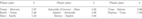Table 1

where $R$ – radius of the planet orbit, $n$ – planetary number, $b$ – empirical parameter. The values of $b$ for different planets are presented in Table 1.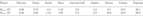Table 2

This equation satisfactorily describes the planetary distances from the Sun if the parameter $b$ is determined separately for outer planets and planets of the Earth's group (Table 2). Futhermore many researchers made attempts to relate distances of planets from the Sun with the process of planetary system formation [Vitjazev et al., 1990].

The planetary distances from the Sun can be accurately described by the logarithmic spiral equation [Anfilogov and Khachay, 2015]:

\begin{equation} \tag*{(1)} R = 0.225(\exp 0.172 \varphi) \end{equation}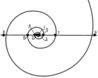Figure 1

where $\varphi$ – angle of the ray from round the spiral polar, $R$ – distance of the planet from the Sun, AU. The points, which represent radiuses of the planet orbits are disposed on the spiral curve on the one line, which passes through the Sun, disposed in the spiral polar. If the Mercury is disposed on the right side of the Sun then the Venus will be disposed on the left side, the Earth on the right and so on, Figure 1. This sequence is broken down for the Jupiter, but the reason is the asteroid belt between the Jupiter and the Mars, Figure 1. The real and calculated values of distances of the planet are represented in Table 2.

It should be noted that the (1) does not describe the planetary space location, only the values of their orbital radiuses. This equation represents the empirical approximation of the relation for distances of planets from the Sun, but it is possible that the spiral alignment of the planet has genetic sense. As a verification of occurrence of the vortical spiral structures at the time of planet formation may be the spiral distribution of the orbital radiuses of four largest Jupiter satellites, which total mass is more than 95% of all satellites mass. This distribution is precisely described by the logarithmic spiral equation:

\begin{equation} \tag*{(2)} R = 330(\exp 0.158 \varphi) \end{equation}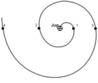Figure 2

where $R$ – satellite orbit radius (thousands km), $\varphi$ – angle of ray torn round the spiral polar Figure 2. It is reasonable safe to suggest that the both the Jupiter and its satellites can be defined as a little autonomic planetary system. Fragment of the spiral arcs revealed in the asymmetrically illuminated disk of MWC 758 [Grady et al., 2013]. The possibility of formation of the big vortical structures in protoplanetary disks is also discussed by Paardekooper et al. .

### 3. The Mass Distribution in the Solar System

An important characteristic of the Solar system is the relationship between the masses of the planets and their distance from the Sun. This relationship for the terrestrial planets is described by the equation of parabola:

\begin{eqnarray*} M_{\mathrm p}/M_{\bigoplus} = - 2.359 R^2 + 4.267R - 1.354 \end{eqnarray*}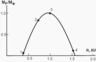Figure 3

where $M_{\mathrm p}$ – mass of the planet, $M_{\bigoplus}$ – mass of the Earth, $R$ – radius of the planet orbit, AU (Figure 3).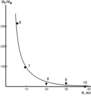Figure 4

Masses of the outer planets are described by the hyperbola equation (Figure 4):

\begin{equation} \tag*{(3)} M_{\mathrm p}/M_{\bigoplus} = 3.9R^{-2.0} \end{equation}

Masses of the terrestrial planets are negligible and they are not situated on this curve. As indicated earlier the chemical composition of these planets is radically different from the composition of outer planets and the Solar system as a whole, but it is unreal that the deficit of mass and different composition of the interior part of the protoplanetary cloud was initially like that. Consequently, the interior planets lost their masses during the process of formation the Sun and the outer planets. The parabolic form of curve (4) indicates that the material from the feeding area of the terrestrial planets was redistributed between the Sun and the Jupiter.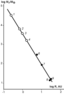Figure 5

We shall attempt to prove this conclusion. The first of all there must be estimated the masses of the terrestrial planets, which has been lost in the process formation. Equation (3) must be transformed to logarithmic form for this purpose. This dependence is shown on Figure 5. Mass of material, which must be at $\log R=0$ can be estimated by extrapolation. This mass has proto-Earth in the initial stage of its formation. The extrapolation gives us magnitude $8 \times 10^3 M_{\bigoplus}$, where $M_{\bigoplus}$ – mass of the Earth. The initial mass, which must be on the Mercury position, is $10^5 M_{\bigoplus}$. This value is commensurable to the mass of the Sun.

Determination of the composition of material lost by inner planets is the next step. We presume that the composition of proto-sun cloud was consistent with the distribution of elements in outer space. The major element in this distribution is hydrogen, which quality equal $2.8 \times 10^8$ atoms per 10,000 atoms Si [Voitkevich et al., 1977]. Let us suppose that hydrogen and helium were the main elements, removed from the inner region of the protoplanetary cloud at the time of the Sun and Jupiter formation.

In order to estimate lost mass of these elements it is necessary to correlate it with the content of the element, retained in the inner planets at the time of their formation. Fe can be selected as an element for correlation. There are $0.67 \times 10^5$ of H and $0.5 \times 10^4$ atoms of He per one atom of Fe in outer space. There is 34.0 mass % Fe in the Earth, or $6.07 \times 10^{-3} M_{\bigoplus}$ g-atoms. The quantity of g-atoms H and He, which must be in primary material of the Earth is equal $1.1 \times 10^3 M_{\bigoplus}$. This value is commensurable with the mass of material, which must be on the distance from the Sun according to (3). It is impossible to explain lost such a big mass of the material by dissipation of volatile elements from atmosphere after the Earth had formed. The calculation presented above favors the assumption that deficit of the mass of the inner planets was caused by removing of their masses to growing Sun and Jupiter.

The problem of the re-distribution of planetary material from the region near 1 AU from the host star appeared after discovery of the planetary systems, having the Jupiter mass planets in this region [Buruteau and Lin, 2010, Matsushita et al., 2013]. There are some models proposing the different variants of migration of the big planets from this region [Alexander and Armitage, 2009]. The variant presented above can be the alternative of these models.

We can suppose that the planetary systems having the "Hot Jupiters" are still on the beginning stage of evolution and the distribution of their mass is described by hyperbola (3). Masses of the big hot planets will be re-distributed between the host star and planets, which forming on distance near 5 AU on the next stage and group of the terrestrial planets can be formed from residual material on the positions of the big planets. It is felt that the major part of re-distributed material will be comprised from hydrogen and helium. Because of that the composition of the terrestrial planets will differ from the central star and outer planets. Possibility of two stage formation of the planetary systems is considered by Alexander and Armitage, .

The active role of the Jupiter in re-distribution of mass of the Solar system is corroborated by availability of gravitation boundary, which position is fixed by asteroid belt. It is believed that it represents the zone of gravitation equilibrium between the Sun and the Jupiter took place at time of their concurrent growth. The position of this zone was not constant. The Sun gravitation attraction grew more quickly, than the Jupiter one. Because of that the equilibrium zone moves in direction from the Earth toward Jupiter and stopped on asteroid belt distance. It is believed that the Mars was in zone of attraction of the Jupiter in time its formation. This allows to explain inverse of the rotation round axis of the Mars and maximum deviation of its orbit radius magnitude from values, determined by (2). Origin of asteroids becomes understandable now. They can be considered as planet embryos, formed on the initial stage and unable to unit into planet because they were in the gravitation equilibrium zone between the Jupiter and the Mars.

### 4. Outstanding Questions

We suppose that peculiarity of alignment of the planets around the Sun and distribution of their mass presented above can be used as the beginning and borderline conditions for development new models of the formation of the planetary systems. We propose three hypotheses, which can be used as a base of these models.

#### Hypothesis 1.

Besides molecular clouds there is another source of material, from which stars and planetary systems were formed. The presence of short-lived isotopes $^{26}$Al, $^{53}$Mn, $^{60}$Fe and $^{180}$Hf in the Solar system is a guide for this assumption. It is assumed that these isotopes were formed at Supernova explosion and then were injected into protosolar cloud [Ouellette et al., 2010, and references therein; Wadhawa et al., 2006, and references therein]. This raises the question: If the short-lived isotopes were really injected into the Solar system there appear a question, where disappear stable isotopes of these elements. Logical deduction is drown that all protosolar cloud could be fully formed from the products of Supernova explosion.

#### Hypothesis 2.

All the present day models of the formation of the planetary system started from assumption that planets had formed from protoplanetary disk which had disposed round the central star. The presence of gas-dust disks round some stars, which have no planets is the base of this assumption. If the Hypothesis 1 is correct, the protosolar cloud has a form of high temperature plasma jet. The electromagnetic forces could play a great role in the process of the central star and planet formation in this situation and rate of this process may be much more than at formation of the planets from gas-dust disk.

#### Hypothesis 3.

The jet of plasma can roll up to the spiral whirl where material moves to the pole of the spiral where the central star forms. Planets may be formed in the points of the spiral, where vortex turbulence appeared as a result of the interaction of gravitational and electromagnetic fields. These hypotheses can be used for construction of the new models of formation of the planet systems.

### References

Alexander, R. D., P. J. Armitage (2009), Giant planet migration, disk evolution and the origin of terrestrial disks, Astrophys. J., 704, p. 989–1001, doi:10.1088/0004-637X/704/2/989.

Anfilogov, V. N., Y. V. Khachay (2015), Some Aspects of the Formation of the Solar System, 75 pp., Springer Briefs in Earth Sciences, Springer Cham Heidelberg New York Dordrecht London.

Buruteau, C., D. N. C. Lin (2010), Protoplanetary migration in turbulent isothermal disk, Astrophys. J., 709, p. 759–773, doi:10.1088/0004-637X/709/2/759.

Grady, C. A., T. Muto, J. Hashimoto (2013), Spiral arms in the asymmetrically illuminated disk on MWC 758 and constraints on giant planets, Astrophys. J., 762, p. 48–60, doi:10.1088/0004-637X/762/1/48.

Hasegawa, Y., S. Ida (2013), Do giant planets survivetype II migration, Astrophys. J., 774, p. 146–154, doi:10.1088/0004-637X/774/2/146.

Matsushita, S., S. Ida, M. Nagasawa (2013), Effect of dinamical evolution of giant planets of survival of terrestrial planets, Astrophys. J., 767, p. 129–142, doi:10.1088/0004-637X/767/2/129.

Moriarty, J., N. Madhusudhan, D. Fischer (2014), Chemistry in an evolution protoplanetary disk effect on terrestrial planet composition, Astrophys. J., 787, p. 81–90, doi:10.1088/0004-637X/787/1/81.

Nagasava, M., E. W. Thommes, S. J. Kenyjn (2006), The diverse origin of terrestrial planet systems, Protostars and Planets, V. B. Reipurth, G. Jewitt and K. Keil (Eds.), p. 639–654, Univ. of Arizona Press, Tucson, AZ.

Ouellette, N., S. N. Desh, J. J. Hester (2010), Injection of Supernova dust in nearby protoplanetary disk, Astrophys. J., 711, p. 597–612, doi:10.1088/0004-637X/711/2/597.

Paardekooper, S.-J., L. Geoffroy, J. S. B. Papalozou (2010), Vortex migration in protoplanetary disks, Astrophys. J., 725, p. 146–158, doi:10.1088/0004-637X/725/1/146.

Papaloizou, J. C. B., et al. (2006), Disk-planet interaction during planet formation, Protostars and Planets, V. B. Reipurth, G. Jewitt and K. Keil (Eds.), p. 655–668, Univ. of Arizona Press, Tucson, AZ.

Vitjazev, A., G. Pechernikova, V. Safronov (1990), The Earth's Group of Planets, 296 pp., Nauka, Moscow.

Voitkevich, G., et al. (1977), Handbook on Geochemistry, 184 pp., Nedra, Moscow (in Russian).

Zarkov, V., V. Trubitsin (1980), Physics of the Earth and Planets, 416 pp., Nauka, Moscow (in Russian).

Wadhawa, M., Y. Amelin, A. M. Davis (2006), From dust to planetesimales: Implication for the Solar protoplanetary disk from short-lived radionuclides, Protostars and Planets, V. B. Reipurth, G. Jewitt and K. Keil (Eds.), p. 835–848, Univ. of Arizona Press, Tucson, AZ.

Received 2 February 2016; accepted 4 February 2016; published 18 February 2016.Citation: Anfilogov V. N. (2016), Re-distribution of the planetary material between the Sun and giant planets at the process of the terrestrial planets formation, Russ. J. Earth Sci., 16, ES1003, doi:10.2205/2016ES000563.

Generated from LaTeX source by ELXpaper, v.1.5 software package.